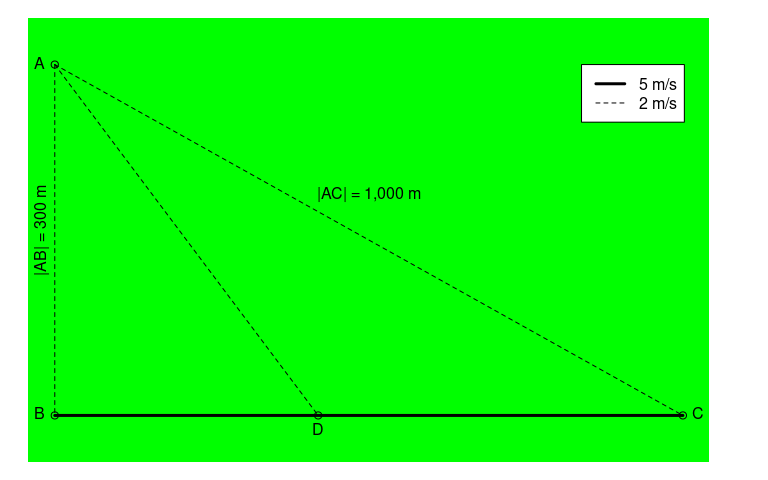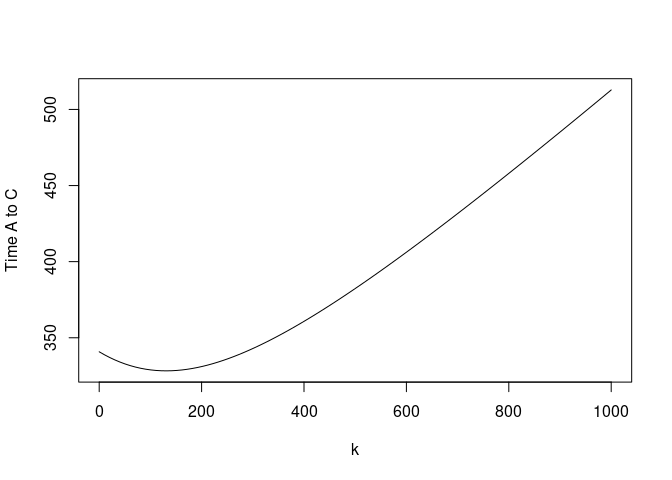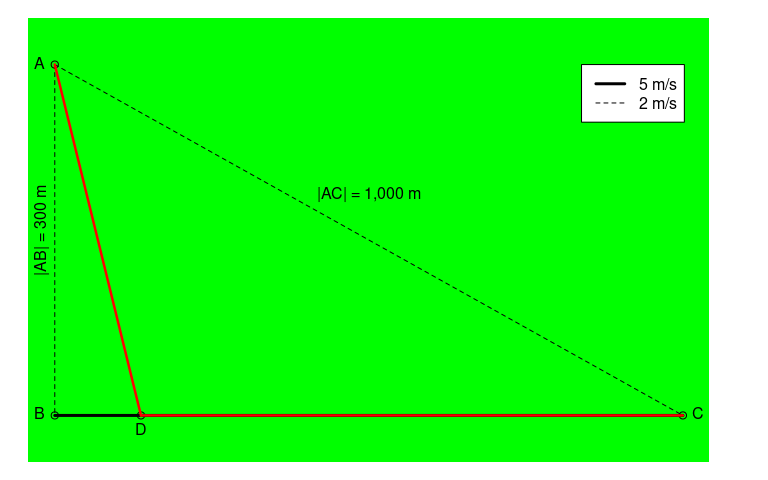# 22 - Fastest route through the forest

library(caracas)

A problem was posted by the Danish newspaper, Ingeniøren, and it goes like this:

You are in the middle of a dense forest located at $$A$$. You need to get to $$C$$ in the fastest way possible, and you can only change direction once. You can walk directly via $$AB$$ to the dedicated walking path $$BC$$ where you can walk fast, you can take the direct path through the forest ($$AC$$) where you have to walk slower, or cross through the forest to the dedicated walking path ($$AD$$ and then $$DC$$).## Information given

• Distances
• $$|AB| =$$ 300 m
• $$|AC| =$$ 1,000 m
• Velocities
• $$v_{AB} = 2$$ m/s
• $$v_{AD} = 2$$ m/s
• $$v_{AC} = 2$$ m/s
• $$v_{BC} = 5$$ m/s

### Length of line segments

We parameterise with $$k = |BD|$$, the distance between $$B$$ and $$D$$. That is, how much to walk on fast walking path before crossing into the forest.

Formulating using caracas:

AB <- as_sym('300')
AB
#> c: 300
AC <- as_sym('1000')
AC
#> c: 1000
BC <- sqrt(AC^2 - AB^2)
BC
#> c: 100⋅√91
k <- symbol('k') # |BD|
DC <- BC - k
#> c:    ____________
#>      ╱  2
#>    ╲╱  k  + 90000

So for a distance of $$|AD|$$, you travel by 5 m/s, and then for a distance of $$- k + 100 \sqrt{91}$$ you travel by 2 m/s. Thus it takes $$\frac{\sqrt{k^{2} + 90000}}{2}$$ to travel $$AD$$ and $$- \frac{k}{5} + 20 \sqrt{91}$$ to travel $$DC$$.

The question is: What is the fastest way to get from $$A$$ to $$C$$?

First, the total duration of the route is:

l <- AD/2 + DC/5
l
#> c:          ____________
#>            ╱  2
#>      k   ╲╱  k  + 90000
#>    - ─ + ─────────────── + 20⋅√91
#>      5          2
lfun <- as_expr(l)
lfun
#> expression(-k/5 + sqrt(k^2 + 90000)/2 + 20 * sqrt(91))
ks <- seq(0, as_expr(AC), length.out = 100)
ls <- eval(lfun, list(k = ks))
plot(ks, ls, type = "l", xlab = "k", ylab = "Time A to C")It looks like a minimum around $$k = 150$$.

We find the analytical solution by first finding critical points:

dl <- der(l, k)
dl
#> c:         k           1
#>    ───────────────── - ─
#>         ____________   5
#>        ╱  2
#>    2⋅╲╱  k  + 90000
crit_points <- solve_sys(dl, k)
crit_points
#> Solution 1:
#>   k =  200⋅√21
#>       ───────
#>          7
best_k <- crit_points[]\$k
best_k
#> c: 200⋅√21
#>    ───────
#>       7

The type of the critical point is found by considering the Hessian:

eval(as_expr(der(dl, k)), list(k = as_expr(best_k)))
#>  0.001283121

Thus the critical point is indeed a minimum as suggested by the plot.

The fastest route is thus obtained for $k = \frac{200 \sqrt{21}}{7} \approx 130.93 .$ It has a length of (in meters)

DC_best <- BC - best_k
#> c: 500⋅√21
#>    ───────
#>       7
best_route
#> c: 300⋅√21
#>    ─────── + 100⋅√91
#>       7
as_expr(best_route)
#>  1150.335

$\frac{300 \sqrt{21}}{7} + 100 \sqrt{91} \approx 1150.34$ and takes (in seconds)

best_l <- subs(l, "k", best_k)
best_l
#> c: 30⋅√21 + 20⋅√91
as_expr(best_l)
#>  328.2651

$30 \sqrt{21} + 20 \sqrt{91} \approx 328.27$

The best route can be illustrated, too: